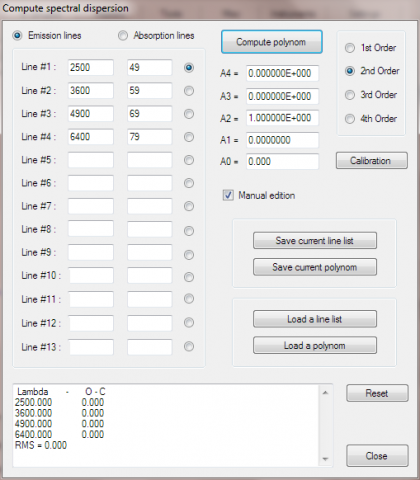# OK I now know what ISIS is doing

Home Forums Spectroscopy Help with ISIS OK I now know what ISIS is doing

#577801

OK I now understand what ISIS is doing when it calculates the coefficients. It adds 1 to all the pixel positions before calculating the fit.  You can see this in the attached trivial example where I manually entered a series of wavelengths and pixel positions where the wavelength = pixel ^2  before making a 2nd order fit. It only gave the expected coefficients when I subtracted 1 from the pixel positions.

I still have no idea why though! (For information VSpec calculates the coefficients conventionally)

Cheers

Robin